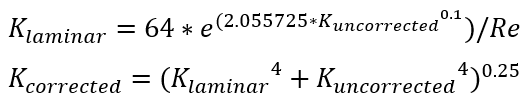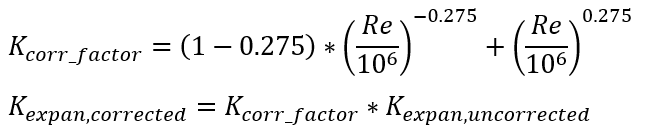# Reynolds Number KLoss Corrections

Flow Simulator elements uses Reynolds number correction factors for various elements. The Kloss (Kuncorrected ) calculated for each element will be corrected to account for Reynolds number using one of the options mentioned below. These correction factors curves are obtained from Internal Flow Systems by D S Miller

1. General Purpose – Recommended for abrupt expansion, abrupt contraction, and valves

The correction factors are obtained from Laminar loss coefficient relationship with turbulent loss coefficient from D.S. Miller Internal Flow Systems 2nd Ed. Fig. 14.32.

Curve fit equations for figure above:2. Diffuser correction & Conical Expansion – Recommended for diffusers (RE_CORR = 2)

The correction factors apply only for expansions and are obtained Reynolds Number correction factors from D.S. Miller Internal Flow Systems 2nd Ed. Fig. 11.7.

Assumption: Outlet section (pipe) exists at the end of the diffuser (not free discharge)

Curve fit equations for figure above: